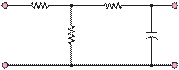### Create an Account

Already have account?

### Forgot Your Password ?

Home / Questions / Repeat Problem 61 for the circuit of Figure P63 d Determine the frequency ω ωC for wh...

# Repeat Problem 61 for the circuit of Figure P63 d Determine the frequency ω ωC for which the imaginary part of the expression in part c is equal to

Repeat Problem 6.1 for the circuit of Figure P6.3.

d.    Determine the frequency ω ωfor which the imaginary part of the expression in part c is equal to 1.

e.    Estimate (without computing it) the magnitude and phase angle of Z( j ω) at ω = 10 rad/s and ω

52,000 Ù                1,000 Ù

+                                                       +

= 10

rad/s.

vin(t)

Figure P6.3

2,000 Ù

10 mF

vout(t)

Jun 18 2020 View more View Less

#### Answer (Solved)Subscribe To Get Solution